# DAV Class 5 Maths Chapter 7 Worksheet 6 Solutions

The DAV Maths Book Class 5 Solutions and DAV Class 5 Maths Chapter 7 Worksheet 6 Solutions of Multiplication and Division of Decimal Numbers offer comprehensive answers to textbook questions.

## DAV Class 5 Maths Ch 7 Worksheet 6 Solutions

Question 1.
Divide the following:
(a) 0.95 ÷ 5
Solution:
0.95 ÷ 5
Here Dividend = 0.95, Divisor = 5
(The decimal point will come directly above the decimal point in the dividend)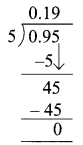Q = 0.19, R = 0

(b) 3.44 ÷ 8
Solution:
3.44 ÷ 8
Here Dividend = 3.44, Divisor = 8
(As the whole number is smaller than the divisor so first decimal will come. Take the number just beside the decimal and divide)Q = 0.43, R = 0(c) 4.9 ÷ 7
Solution:
4.9 ÷ 7
Here Dividend = 4.9, Divisor = 7
(First decimal will come then divide)Q = 0.7, R = 0

(d) 25.41 ÷ 11
Solution:
25.41 ÷ 11
Here Dividend = 25.41, Divisor = 11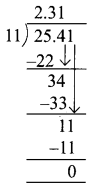Q = 2.31, R = 0

(e) 31.5 ÷ 9
Solution:
31.5 ÷ 9
Here Dividend = 31.5, Divisor = 9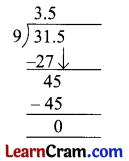Q = 3.5, R = 0(f) 16.5 ÷ 15
Solution:
16.5 ÷ 15
Dividend = 16.5
Divisor = 15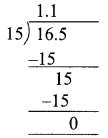Q = 1.1; R = 0

(g) 0.077 ÷ 7
Solution:
0.077 ÷ 7
Dividend = 0.077
Divisor = 7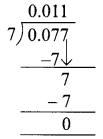Q = 0.01; R = 0

(h) 88.88 ÷ 22
Solution:
88.88 ÷ 22
Dividend = 88.88
Divisor = 22Q = 4.04, R = 0(i) 35.49 ÷ 13
Solution:
35.49 ÷ 13
Dividend = 35.49
Divisor =13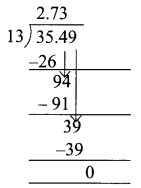Q = 2.73, R = 0

(j) 57.5 ÷ 25
Solution:
57.5 ÷ 25
Dividend = 57.5
Divisor = 25Q = 2.3, R = 0DAV Class 5 Maths Chapter 7 Worksheet 6 Notes

Division of decimal number by a whole number.

Example.
Divide 20.28 by 6
Solution:
The decimal point will come directly above the decimal point in the dividend
Dividend = 20.28,
Divisor = 6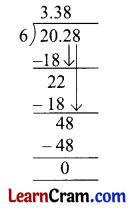Quotient = 3.38, Remainder = 0